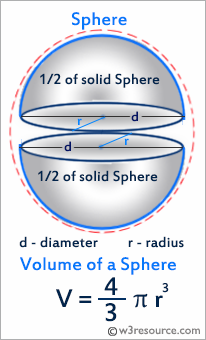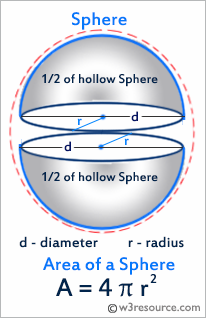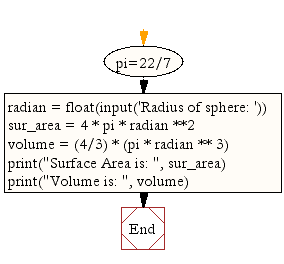﻿ Python Math: Calculate surface volume and area of a sphere - w3resource# Python Math: Calculate surface volume and area of a sphere

## Python Math: Exercise-6 with Solution

Write a Python program to calculate surface volume and area of a sphere.
Note: A sphere is a perfectly round geometrical object in three-dimensional space that is the surface of a completely round ball.

Python: Surface volume and area of a sphere

A sphere is a perfectly round geometrical object in three-dimensional space that is the surface of a completely round ball.

In three dimensions, the volume inside a sphere is derived to be V = 4/3*π*r3 where r is the radius of the sphereThe  area of a sphere is A = 4*π*r2Sample Solution:-

Python Code:

``````pi=22/7
sur_area = 4 * pi * radian **2
volume = (4/3) * (pi * radian ** 3)
print("Surface Area is: ", sur_area)
print("Volume is: ", volume)
```
```

Sample Output:

```Radius of sphere: .75
Surface Area is:  7.071428571428571
Volume is:  1.7678571428571428
```

Flowchart:## Visualize Python code execution:

The following tool visualize what the computer is doing step-by-step as it executes the said program:

Python Code Editor:

Have another way to solve this solution? Contribute your code (and comments) through Disqus.

What is the difficulty level of this exercise?

Test your Programming skills with w3resource's quiz.

﻿

## Python: Tips of the Day

Clamps num within the inclusive range specified by the boundary values x and y:

Example:

```def tips_clamp_num(num,x,y):
return max(min(num, max(x, y)), min(x, y))
print(tips_clamp_num(2, 4, 6))
print(tips_clamp_num(1, -1, -6))
```

Output:

```4
-1
```